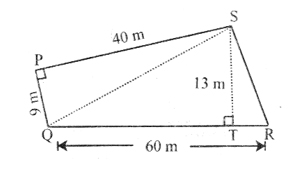# SSC Worksheet for Chapter - 3 Area Class 8

## Find SSC Worksheet for chapter-3 Area class 8

CLASS-8

BOARD: SSC

Mathematic Worksheet - 3

TOPIC: Area

For otherSSC Worksheet for class 8 Mathematiccheck out main page of Physics Wallah.

1. The area of parallelogram whose base is 12cm and height 7cm is
1. 34
2. 64
3. 74
4. 84
2. The area of a parallelogram is 56 sq cm and its height is 7cm. Then its base is
1. 4
2. 6
3. 8
4. 10
3. The area of a parallelogram is 390 sq cm. If its height is 26cm. Then its base is
1. 10
2. 15
3. 20
4. 25
4. The area of triangle whose base is 18.2 cm and height, 7.5 cm is
1. 68.25
2. 69.50
3. 70.25
4. 72.75
5. The sides of a right angled triangle forming the right angle are 16cm and 8cm. What is its area?
1. 64
2. 74
3. 84
4. 94
6. A triangle has an area of 11.6 sq cm. If its height is 2.9cm, what is its base?
1. 6
2. 8
3. 10
4. 12
7. The diagonals of a rhombus are 84 cm and 42 cm long. What is the area of the rhombus?
1. 1764
2. 1294
3. 968
4. 3214
8. The length of the diagonal of a rhombus are 12 cm and 18cm. What is the area of the rhombus.
1. 202
2. 96
3. 108
4. 162
9. The area of a rhombus is 702 sq cm. If one of its diagonals is 54 cm long, what is the length of the other?
1. 24
2. 14
3. 16
4. 26
10. The length of the parallel sides of a trapezium are 8.7 cm and 5.3 cm. If the perpendicular distance between them is 4.5cm, what is its area?
1. 19.25
2. 31.5
3. 17.5
4. 32.5
11. The area of a trapezium is 262.5sq cm and the perpendicular distance between its parallel sides is 15cm,then what is the sum of its parallel sides?
12. The area of a trapezium is 84.5 sq cm and its height is 6.5cm. If one of its parallel sides is 15.2cm long, the length of the other side is
13. The∠ PQRSalongside is the map of a plot of land. Using the given measures, find its area.
14. If the length of the sides of a triangular plot of land are 20m, 21m and 13m, what is its area?### Solutions: to worksheet-3 Topic-Area

 1. (d) 2. (c) 3. (b) 4. (a) 5. (a) 6. (b) 7. (a) 8. (c) 9. (d) 10. (b)